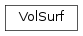# mvpa2.misc.surfing.volsurf.VolSurf¶class `mvpa2.misc.surfing.volsurf.``VolSurf`(vg, white, pial, intermediate=None)

Associates a volume geometry with two surfaces (pial and white).

Attributes

 `intermediate_surface` Returns the node-wise average of the pial and white surface `pial_surface` Returns the pial surface `volgeom` Returns the volume geometry `white_surface` Returns the white surface

Methods

 `coordinates_to_grey_distance_mm`(nodes, xyz) Computes the grey position of coordinates in metric units `surf_project_nodewise`(xyz) Projects coordinates on lines connecting pial and white matter. `surf_project_weights`(nodes, xyz) Computes relative position of xyz on lines from pial to white matter. `surf_project_weights_nodewise`(xyz) Computes relative position of xyz on lines from pial to white matter. `surf_unproject_weights_nodewise`(weights) Maps relative positions in grey matter to coordinates
Parameters: volgeom: volgeom.VolGeom Volume geometry white: surf.Surface Surface representing white-grey matter boundary pial: surf.Surface Surface representing pial-grey matter boundary intermediate: surf.Surface (default: None). Surface representing intermediate surface. If omitted it is the node-wise average of white and pial. This parameter is usually ignored, except when used in a VolSurfMinimalLowresMapping.

Notes

‘pial’ and ‘white’ should have the same topology.

Attributes

 `intermediate_surface` Returns the node-wise average of the pial and white surface `pial_surface` Returns the pial surface `volgeom` Returns the volume geometry `white_surface` Returns the white surface

Methods

 `coordinates_to_grey_distance_mm`(nodes, xyz) Computes the grey position of coordinates in metric units `surf_project_nodewise`(xyz) Projects coordinates on lines connecting pial and white matter. `surf_project_weights`(nodes, xyz) Computes relative position of xyz on lines from pial to white matter. `surf_project_weights_nodewise`(xyz) Computes relative position of xyz on lines from pial to white matter. `surf_unproject_weights_nodewise`(weights) Maps relative positions in grey matter to coordinates
`coordinates_to_grey_distance_mm`(nodes, xyz)

Computes the grey position of coordinates in metric units

Parameters: nodes: int or np.ndarray Single index, or Q indices of nodes relative to which the coordinates are computed. If True then grey distances are computed node-wise. xyz: Px3 array with coordinates, assuming ‘white’ and ‘pial’ surfaces have P nodes each. grey_position_mm: np.ndarray Vector with P elements (if type(nodes) is int) or PxQ array (with type(nodes) is np.ndarray) containing the signed ‘distance’ to the grey matter. Values of zero indicate a node is within the grey matter. Negative values indicate that a node is ‘below’ the white matter (i.e. farther from the pial surface than the white surface), whereas Positive values indicate that a node is ‘above’ the pial matter.
`intermediate_surface`

Returns the node-wise average of the pial and white surface

Returns: intermediate: surf.Surface
`pial_surface`

Returns the pial surface

Returns: pial: surf.Surface
`surf_project_nodewise`(xyz)

Projects coordinates on lines connecting pial and white matter.

Parameters: xyz: numpy.ndarray (float) Px3 array with coordinates, assuming ‘white’ and ‘pial’ surfaces have P nodes each xyz_proj: numpy.ndarray (float) Px3 array with coordinates the constraints that xyz_proj[i,:] lies on the line connecting node ‘i’ on the white and pial surface, and that xyz_proj[i,:] is closest to xyz[i,:] of all points on this line.
`surf_project_weights`(nodes, xyz)

Computes relative position of xyz on lines from pial to white matter.

Parameters: nodes: True or np.ndarray or int Q node indices for each the weights are computed. If True, then weights are computed node-wise, otherwise separately for each node. xyz: numpy.ndarray (float) Px3 array with coordinates. IF nodes is True then the ‘white’ and ‘pial’ surfaces must have P nodes each. weights: numpy.ndarray (float) If nodes is True, P values of relative grey matter positions (0=white surface and 1=pial surface), where the i-th element are the projection weights for xyz[i] relative to the i-th node in the pial and white surface. Otherwise it returns an PxQ array with the projected weights for each node. If nodes is an int, then a P-vector is returned
`surf_project_weights_nodewise`(xyz)

Computes relative position of xyz on lines from pial to white matter.

Parameters: xyz: numpy.ndarray (float) Px3 array with coordinates, assuming ‘white’ and ‘pial’ surfaces have P nodes each. weights: numpy.ndarray (float) If nodes is True, P values of relative grey matter positions (0=white surface and 1=pial surface), where the i-th element are the projection weights for xyz[i] relative to the i-th node in the pial and white surface. Otherwise it returns an PxQ array with the projected weights for each node. If nodes is an int, then a P-vector is returned
`surf_unproject_weights_nodewise`(weights)

Maps relative positions in grey matter to coordinates

Parameters: weights: numpy.ndarray (float) P values of relative grey matter positions, where 0=white surface and 1=pial surface. xyz: numpy.ndarray (float) Px3 array with coordinates, assuming ‘white’ and ‘pial’ surfaces have P nodes each.
`volgeom`

Returns the volume geometry

Returns: vg: volgeom.VolGeom
`white_surface`

Returns the white surface

Returns: white: surf.Surface